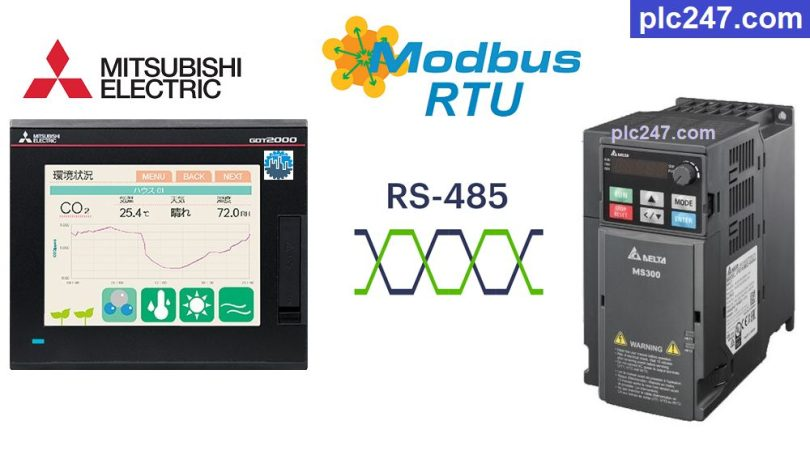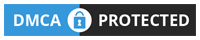# Mitsubishi HMI “Modbus RTU” Delta MS300 TutorialWritten by

The MS300 Series Delta inverters are a compact line of vector controllers that are rugged, compact, and compact in design. It also has 1 built-in USB port and 1 RS485 port to support remote control by Modbus RTU.

Today plc247.com will guide you to use HMI Mitsubishi GT2505-VTBD to control Delta MS300 inverter through Modbus-RTU protocol.

#### Mitsubishi GT2505 & Delta MS300 “Wiring Diagram”

Below is the connection diagram according to RS485 communication standard between HMI GT2505-VTBD and Delta MS300 inverter

#### Delta MS300 “Modbus RTU” Parameters Setting

To be able to control Delta MS300 with Modbus-RTU, in addition to the basic parameters of the inverter (frequency, current, voltage…) we need to install the following communication parameters:

+ P00.20 = 1 (Using Modbus Setting Frequency)

+ P00.21 = 2 (Using Modbus Control Motor)

+ P09.00 = 1 (Slave Address = 1)

+ P09.01 = 9.6 (Baudrate = 9600bps)

+ P09.02 = 3

+ P09.03 = 1.0 (Overtime Com. = 1.0s)

+ P09.04 = 14 ( Modbus-RTU 8/E/1 Selection)

##### Note: Mitsubishi GOT2000 Holding Registers Address = “VFD Address (Dec) + 400001“

>>> Motor Control Address = 2000 (hex) = 8192 (dec) + 400001 = 408193 (dec)

• Set Word 408193 = 18 (dec): Forward Run
• Set Word 408193 = 34 (dec): Reverse Run
• Set Word 408193 = 1 (dec): Stop

>>> Setting Frequency Address = 2001 (hex) = 8193 (dec) + 400001 = 408194 (dec)

• Output Frequency Address = 2103 (hex) = 8451 (dec) + 400001 = 408452 (dec)
• Output Current Address = 2104 (hex) = 8452 (dec) + 400001 = 408453 (dec)
• Output Volatge Address = 2106 (hex) = 8454 (dec) + 400001 = 408455 (dec)

#### Mitsubishi GT2505-VTBD Modbus Configuration

You need to install Modbus communication parameters for Mitsubishi GT2505-VTBD exactly the same as those installed for Delta MS300 inverters

### HMI Programming Example

+ Main Control Panel

+ Frequency Setting

+ Forward Run Command

+ Reverse Run Command

+ Stop Run Command

+ Output Frequency Monitoring

+ Output Current Monitoring

+ Output Voltage Monitoring

### Project Video Tutorial

======

References